top of page# Suppose a vector V is transformed by matrix M1  to generate vector VT.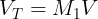# ​Further VT is transformed by M2to generate vector VTT.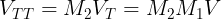# ​If V is transformed by Mto generate vector VTT directly.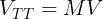# Here transformation M is same as combined effect of transformation M2  performed after transformation M1. M is referred as multiplication of matrices M1  and M2 .# Suppose matrix M1  and M2  are: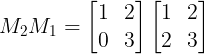# First and second column of matrix M1 represents transformation of unit vector i and j respectively. Further when transformation M2 is performed, overall effect of the two will change the unit vectors to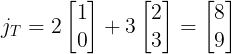# iT and jT represent first and second column respectively of overall transformation matrix i.e. product matrix of M1 and M2.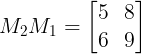# This way, direction of transformation remain same but magnitude gets multiplied by k times.• # Multiplication of matrix is not commutative, since applying transformation M1 after M2 is not same as applying transformation M2 after M1.• # Matrix multiplication is associative.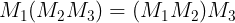• # Transpose of product shows following relation:bottom of page